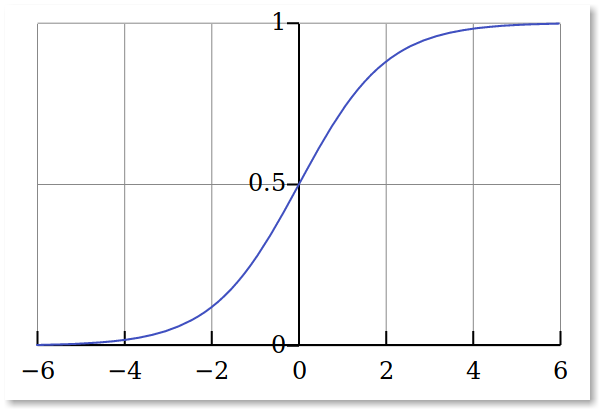When it comes to machine learning, there is a no free lunch theorem, which states the fact that no one algorithm functions best for every problem.

As an example, you cannot state that neural networks are usually better than decision trees or vice-versa. Hence, you should try a bundle of different algorithms for your problem in order to properly establish performance and which algorithm works best for your case.

Related course:

### Algorithms

Let`s see what are the top 10 machine learning algorithms you should try.

1. Linear Regression is one of the most popular machine learning algorithms used for statistics. The core principle of this algorithm is the fact that it depicts the relationship between the input variables and the output variables, by identifying certain weightings for the input variables called coefficients.

2. Logistic Regression is great for binary classification problems, with the purpose of using a logistic function to identify values for the coefficients that weight each input variable.1. Linear discriminant analysis is used for more than two class classification problems and it consists of statistical properties of your data, established for each class. Predictions are done by measuring a discriminate value for each class and by making a prediction for the class with the biggest value.

2. Decision Trees represent a type of algorithm useful for predictive modelling, while their representation model is a binary tree. Each node of the tree stands for a single input variable and a split point on the variable. The leaves contain the output variables that aid in making a prediction. This algorithm is easy and fast to use, usually being helpful for a multitude of problems due to the fact that it does not require any particular preparation for your data.

3. Naïve Bayes is a popular algorithm used for predictive modelling, being called naïve because of the fact that it presumes that each input variable is independent. This algorithm is great for complex problems.

4. K-Nearest Neighbors is an algorithm for which the predictions are done for a new data set by searching through the entire training set for the K most similar neighbors, while summing up the output variable for those K instances. It is useful for both regression and classification problems, but KNN can require a lot of space to store all the data needed.

5. The Learning Vector Quantization represents an artificial neural network algorithm that permits you to choose how different training instances hang onto, while learning exactly what those instances should look like. Thus, if you come to a situation in which the KNN provides good results based on your dataset, you can opt for using LVQ to diminish the storing requirements of your data.

6. Support Vector Machines are one of the most popular amongst machine learning algorithms. It is considered an out-of-the box classifier that uses a hyperplane in order to obtain the best separate points in the input variable space by their class.

7. The Random Forest algorithm is also called the Bootstrap Aggregation, referring to a statistical manner for estimating quantity from a data sample.

8. Boosting represents a method that tries to establish a strong classifier from a number of weak classifiers. This is achieved by setting up a model from the training data, while creating a second model meant to correct the errors that appear in the first model.

The algorithms mentioned above are the most popular when it comes to machine learning, being used in relation to each other depending on the type of problem you aim to solve. However, even an experienced data scientist cannot state which algorithm is the best before actually experimenting with different algorithms.